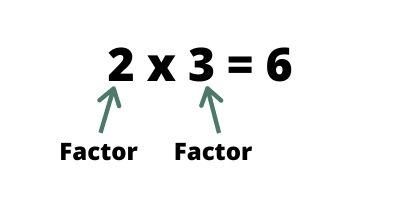# Factors of 30

## What is the factor?

Factors are integers you multiply together to get another integer. Also remember that the factors always include 1 and itself.NOTE: When finding the factors of a number, ask yourself “what numbers can be multiplied together to give me this number?.

## How to find the factors of 30?

THINK: What pairs of numbers can be multiplied together to give me 30?

Step 1: 1 x 30 = 30, so put these numbers in the factor list.

 1 … 30

Step 2: Take 2 and divide with 30. It gives 2 x 15 = 30.

 1 2 15 30
Step 3:Take 3 and divide with 30. It gives 3 x 10 = 30
 1 2 3 10 15 30

Step 4: Take 4 and divide with 30. The remainder will be 2. But factors always give 0 remainder. This means 4 is NOT a factor of 30.

Step 5: Take 5 and divide with 30. It gives 5 x 6 = 30.
 1 2 3 5 6 10 15 30
Step 6: Take 6 and divide with 30. It gives 6 x 5 = 30. but factors 6 and 5 are already in the list. So you dont need to repeat the number.
 1 2 3 5 6 10 15 30

Step 7: Take 7 and divide with 30. The remainder will be 2. But factors always give 0 remainder. This means 7 is NOT a factor of 30.

Step 8: Take 8 and divide with 30. The remainder will be 6. But factors always give 0 remainder. This means 8 is NOT a factor of 30.

Step 9: Take 9 and divide with 30. The remainder will be 3. But factors always give 0 remainder. This means 9 is NOT a factor of 30.

Step 10: Take 10 and divide with 30. It gives 10 x 3 = 30. but factors 10 and 3 are already in the list. So you dont need to repeat the number.

 1 2 3 5 6 10 15 30

So the factors of 30 are 1, 2, 3, 5, 6, 10, 15, 30.

TIPS: Try to keep tables (at least till 20) on your finger tips. Revise tables every day## Is 30 a prime or composite number?

30 is a composite number not prime number because it has more than 2 factors.

What is composite number? – The composite numbers are the positive integers which have more than two factors.

What is prime number? – An integer number that is divisible by two distinct integers, 1 and itself.

## What is the prime factorization of 30

The prime factorization of 30 are  2 x 3 x 5

## Factor pairs of 30

Factors are often given as pairs of numbers, which multiply together to give the original number. These are called factor pairs

What is factor pairs: Factor pairs are combinations of two factors that multiply together to give the original number.

Factor pairs of 30 are:
1 x 30 = 30
2 x 15 = 30
3 x 10 = 30
5 x 6 = 30
6 x 5 = 30
10 x 3 = 30
15 x 2 = 30
30 x 1 = 30

## Is 30 a square number?

No, 30 is NOT a square number.
The square root of 18 is 5.48.
The square of 30 is 900.

Hope you learned to solve the factors of 30. Now try to find out the factors of the following numbers by yourself. FACTORS OF 30 – 1, 2, 3, 5, 6, 10, 15, 30. Apart from factors of 30 you can find another factors below.

Factors of 11
Factors of 18
Factors of 24
Factors of 28
Factors of 30
Factors of 32
Factors of 36
Factors of 40
Factors of 42
Factors of 45
Factors of 48
Factors of 56
Factors of 72

Translate »
Scroll to Top